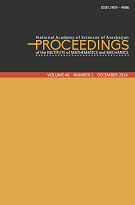P R O C E E D I N G S
of the
Institute of Mathematics and Mechanics

 ISSN 2409-4986 (Print) ISSN 2409-4994 (Online)

## Volume 41, Issue 1

On properties of the generalized Besov-Morrey spaces with dominant mixed derivatives
by Alik M. Najafov and Aygun T. Orujova
315
Inverse Scattering Problem for a Piecewise Continuous Sturm-Liouville Equation with Eigenparameter Dependence in the Boundary Conditon
by Khanlar R. Mamedov, Nida P. Kosar and F. Ayca Cetinkaya
1624
On a special class of semi-cotangent bundle
by Furkan Yıldırım
2538
Constructing extremal elements in approximation by sums of univariate functions
by Arzu M.-B. Babayev and Ibrahim K. Maharov
3943
Boundedness of the multilinear fractional integral operators with rough kernel on Morrey spaces
by Emin V. Guliyev, Amil A. Hasanov and Zaman V. Safarov
4455
On the properties of \$Q\$- and \$Q'\$-integrals of the function measurable on the real axis
by Rashid A. Aliev
5662
A boundary value problem for elliptic-differential operator equations with a spectral parameter in the equation and in the boundary conditions
by Nargul K. Kurbanova
6376
Multi-sublinear rough fractional maximal operator on product modified Morrey spaces
by Sabir G. Hasanov
7787
Trajectories that have points at infinity as limit sets for dynamical systems on the plane
by Maxim V. Shamolin
8893
On behavior at infinity of the solutions of a semilinear elliptic equation of second order
by Shirmail G. Bagirov
94103
Finiteness of the negative spectrum of the one-dimensional magnetic Schrödinger operator
104112
Necessary optimality conditions of quasi-singular controls in optimal control
by Misir J. Mardanov and Kamil B. Mansimov
113122
Coercive estimate for degenerate elliptic parabolic equations
by Tahir S. Gadjiev and Mehriban N. Kerimova
123134
Spectral properties for the equation of vibrating beam
by Ziyatkhan S. Aliyev and Sevinc B. Guliyeva
135145
Alternating algorithm for the approximation by sums of two compositions and ridge functions
by Vugar E. Ismailov
146152
Kazim Hasanov — 80 153156
 © 2022 Institute of Mathematics and Mechanics